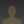0%
0%

Thermistor with arduino to measure temperature

It is an easy way to measure the exact temperature with a thermistor.

Similar projects worth following
215 views
In this project, we have come up with thermistor interfacing with Arduino to measure temperature and display it on the LCD.

Thermistor

The important segment in this circuit is Thermistor, which has been utilized to recognize the rise in temperature. The thermistor is temperature sensitive resistor, whose resistance modifies as per the temperature.

There are two different types of thermistor NTC and PTC, we have used as an NTC type thermistor. NTC thermistor is a resistor whose resistance diminishes as arise in temperature while in PTC it will improve the resistance as arise in temperature.

Calculating Temperature using Thermistor

Vout= (Vin * Rt) / (R + Rt)

So, for estimating the temperature we require the value of thermistor resistance only.

• 1 × NTC thermistor 10k
• 1 × Arduino UNO & Genuino UNO
• 1 × Resistor 10k ohm
• 1 × LCD - 16x2
• 1 × Connecting wires

• 1
Run a Program

#include
#include "LiquidCrystal.h"
LiquidCrystal lcd(44,46,40,52,50,48);
float A = 1.009249522e-03, B = 2.378405444e-04, C = 2.019202697e-07;
float T,logRt,Tf,Tc;
float Thermistor(int Vo) {
logRt = log(10000.0*((1024.0/Vo-1)));
T = (1.0 / (A + B*logRt + C*logRt*logRt*logRt)); // We get the temperature value in Kelvin from this Stein-Hart equation
Tc = T - 273.15; // Convert Kelvin to Celcius
Tf = (Tc * 1.8) + 32.0; // Convert Kelvin to Fahrenheit
return T;
}

void setup(){
lcd.begin(16,2);
lcd.clear();
}

void loop()
{
lcd.setCursor(0,0);
lcd.print("Temp:");
lcd.print("k ");

lcd.setCursor(0,1);
lcd.print((Tc));
lcd.print(" C ;");

lcd.setCursor(9,1);
lcd.print((Tf));
lcd.print(" F");
delay(800);
}

Share

Similar Projects

Project Owner ContributorCatherine Sui